Next: How to install ALIAS Up: ALIAS-C++ Previous: Parser, Generic Solver and

• La page de J-P. Merlet
• La page Présentation de HEPHAISTOS
• La page "Présentation" de l'INRIA

Subsections

# Parallelizing ALIAS programs

Interval analysis has a structure that is appropriate for a distributed implementation. Indeed the principle of the algorithm is to process a list of boxes, the processing for a box being independent of the other boxes in the list (except that it may have be stopped). A classical distributed implementation will rely on the master/slave paradigm. A master computer will manage the list of boxes and monitor the activity of the slaves. As soon as a slave is free the master will send a box to this slave. The slave, which has its own list of boxes, will perform a few iteration of the solving algorithm and then send back to the master the remaining boxes to be processed in its list and eventually the solutions it has found. After receiving a signal from the slave the master will receive the boxes from the slave and append them to its list of boxes. This slave being now free the master will send him another box in its list.

Hence most of the calculation will be performed by the slaves. It may happen however that at a given time all the slaves are busy processing boxes. The master itself may then start a few iteration of the solving algorithm on the current box of its list while carefully monitoring the activity of the slaves.

The structure of the interval analysis algorithms is very convenient for using the above paradigm:

• few data are to be transmitted by the master to the slave: typically for a problem with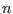unknowns the master will have to send 2floating numbers that describe a box. It may however be interesting to send other information such as the derivatives but still the amount of data will be low
• the processing time of a box is in general much more large than the transmission time

The gain in computation time will in general be lower than the number of used slaves. This is due to

• the transmission time between the master and the slaves
• an overhead of the slave program: in our implementation the slaves uses basically the same program than the master and perform some coherence checks on the unknowns, equations, available memory size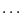before starting the processing of the box
• the fact that only one computer manages the list of boxes. This master may be still managing the boxes send by a slave while others slaves have already finished their processing. Clearly the master/slave paradigm exposed above may not be the best for a large number of computers. In a next version we intend to propose another paradigm for the use of the interval analysis algorithms in the framework of grid computing
Note however that in some cases the gain may be larger than the number of slaves. This is, for example, the case of the optimization algorithms as the independence of box processing is no more true: the discovery of a good optimum by a slave may leads to stop the processing of the other slaves.

In any case a key point of a distributed implementation is to be able to

• stop an algorithm that is run by a slave computer after it has performed some processing on a box
• recover the current state of the slave process i.e. the solutions if any and the remaining unprocessed boxes.

# Stopping a procedure and miscellaneous utilities

There are different methods to stop the current bisection process:

1. the number of boxes still to be processed is greater than a given threshold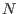: this is obtained by setting the global variable ALIAS_Parallel_Max_Bisection to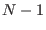. . A safety procedure may be used as the number of bisection may be large before getting the right number of boxes (for example when using the single bisection mode): the maximal number of bisection may be indicated by setting the global variable ALIAS_Parallel_Max_Split and the procedure will return if this number is reached and the number of unprocessed boxes is lower than ALIAS_Parallel_Max_Bisection (otherwise the process will continue until this number is reached).
2. the number of performed bisection is greater than a given threshold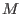: this is obtained by setting the global variable ALIAS_Parallel_Max_Bisection to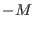3. the number of performed bisection is greater than a given thresholdand the number of boxes still to be processed is lower than a given threshold: this is obtained by setting the global variable ALIAS_Parallel_Max_Bisection toand the global variable ALIAS_Parallel_Max_Box to. A safety mechanism is enforced in that case to avoid that only one of the slave computer will perform all the computation: if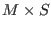bisections have been done the procedure will return the error code -1. The value of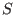is given by the global variable ALIAS_Safety_Factor with a default value of 2
4. if we are using the reverse storage mode we may indicate that the number of performed bisection must be greater than a given thresholdand the number of boxes still to be processed is lower than a given threshold: this is obtained by setting the global variable ALIAS_Parallel_Max_Bisection toand the global variable ALIAS_Parallel_Max_Reverse to5. the slave computation time has exceeded a fixed amount of time, which probably indicate that it will be preferable to distribute the treatment of the processed box among different slaves. This could be done using the double ALIAS_TimeOut which indicates the maximum amount of time (in minutes) during which a slave may run (this amount will be respected only approximatively).
The number of the box still to be processed may be determined from the integer array
ALIAS_Parallel_Box. Let ALIAS_Parallel_Box=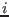and ALIAS_Parallel_Box=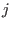. If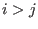, then no boxes remains to be processed otherwise the box numbers to be processed start atand finish at(hence there are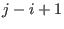boxes to be processed). The boxes are stored in the interval matrix array Box_Solve_General_Interval. If the system hasunknowns, the box for each unknown in the box numberedare given by
Box_Solve_General_Interval(i,1)Box_Solve_General_Interval(i,n). For procedures involving the gradient of the functions you may retrieve the simplified gradient in the integer matrix
Gradient_Solve_General_Interval while for the procedures involving the gradient and hessian of the functions the gradient may be retrieved in the interval matrix Gradient_Solve_JH_Interval. Note that this storage may not be available if you have set the flag ALIAS_Store_Gradient to 0 (its default value is 1).

The variable ALIAS_Parallel_Slave should be set to 0 for a sequential use of the algorithm, to 1 if the algorithm is run by a master computer and to 2 if the algorithm is run by a slave computer.

Using this stop criteria we may implement a parallel version of the ALIAS procedures, see the ALIAS-Maple manual.

If the master/slave scheme is used the master may perform some steps of a solving algorithm but for efficiency purpose it is necessary that the master monitor the slaves to determine if one has completed its calculation. This is the role of the ALIAS_Slave_Returned procedure that stops the processing done by the master as soon as a slave is free. Such a procedure may be found in the lib_Slave.a library: it is based on the pvm message passing mechanism (see the ALIAS-Maple manual). For the non parallel processing the equivalent procedure may be found in the lib_No_Slave.a library. Hence according to a sequential or a parallel use one of this library has to be linked to the main program.

For solving procedures involving the use of the gradient we may use two schemes:

• the master transmits to the slave both the boxes and the simplified gradient
• the master transmits to the slave only the boxes and the slave will first compute the simplified gradient before running the solving algorithm
In the first case the transmission time of the gradient may be relatively large and the master may spend a large amount of time for this transmission while other slaves are currently free. Hence it may be interesting to have a flag which indicate which mode is used: this is the role of the integer ALIAS_Transmit_Gradient.

The ALIAS-Maple library offers a parallel implementation of some solving algorithm. Communication between the slaves and the master is ensured by the message passing mechanism pvm. See the ALIAS-Maple manual for a detailed account of the distributed implementation.

This implementation uses some specific C++ procedures.


void ALIAS_Prepare_Buffer(int DimVar,INTERVAL_VECTOR &X,char *buffer)

which put in the string buffer the lower and upper bounds of the DimVar ranges contained in the interval vector X.

The master and slaves exchange strings and in the parallel implementation offered currently by ALIAS all data are contained in these strings (this may change in the near future as PVM offers other possibility). Each numerical data is introduced by few control character. The following control character are used:

• B: the next floating point numbers will be a box that is sent back by the slave
• BS: same as B except that the box may be used as a parameter by the slave (for example the location of the current optimum)
• F: is followed by an integer. It's used by the slave to indicate how many solutions it will send to the master
• N: just used to indicate that a slave has completed its task and has nothing to send back to a slave
• P: followed by the control character I or F. Its allow to transmit to the slave an integer or a float that will be used as a parameter
• S: the next floating point numbers will be a solution box that is sent back by the slave
• SP: followed by an integer. It's used by the master to indicate to the slave the value of the parameter ALIAS_Parallel_Max_Bisection

Using this convention we may used the following procedure to decode the string sent by the master or the slave:


int Nb_Var, int Nb_Eq,INTERVAL_MATRIX &Box,int *Nb_Total,
INTERVAL_MATRIX &Sol,int *Nb_Sol,int *Nb_Split,
INTEGER_VECTOR &IPar,int *Nb_Ipar,
VECTOR &FPar,int *Nb_Fpar,INTERVAL_MATRIX &BoxPar,
int *Nb_BoxPar)

where mess is the string and
• Box: the Nb_Total boxes with the control character B
• Sol: the Nb_Sol boxes with the control character S
• Nb_Split: the integer following F
• IPar: the Nb_Ipar integer following the control character P I
• FPar: the Nb_Fpar float following the control character P F
• BoxPar: the Nb_BoxPar parameter boxes following the control character BS

If is necessary we may transmit also the simplified jacobian associated to the boxes using the procedure:


int Nb_Var, int Nb_Eq,INTERVAL_MATRIX &Box,
INTEGER_MATRIX &GBox,int *Nb_Total,
INTERVAL_MATRIX &Sol,int *Nb_Sol,int *Nb_Split,
INTEGER_VECTOR &IPar,int *Nb_Ipar,
VECTOR &FPar,int *Nb_Fpar,INTERVAL_MATRIX &BoxPar,
int *Nb_BoxPar)


Here the boxes followed by the simplified jacobian GBox are introduced by the control character BG.

If the true jacobian has to be transmitted we use:


int Nb_Var, int Nb_Eq,INTERVAL_MATRIX &Box,
INTERVAL_MATRIX &GBox,int *Nb_Total,
INTERVAL_MATRIX &Sol,INTEGER_VECTOR &Is_Kanto,
int *Nb_Sol,int *Nb_Split,
INTEGER_VECTOR &IPar,int *Nb_Ipar,
VECTOR &FPar,int *Nb_Fpar,INTERVAL_MATRIX &BoxPar,
int *Nb_BoxPar)

Here the boxes followed by the jacobian GBox are introduced by the control character BG. Furthermore each solution is followed by an integer that indicates the type of the solution and can be accessed by Is_Kanto.Next: How to install ALIAS Up: ALIAS-C++ Previous: Parser, Generic Solver and